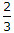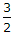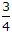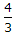# Civil Engineering - Theory of Structures - Discussion

### Discussion :: Theory of Structures - Section 1 (Q.No.27)

27.

The ratio of maximum shear stress to average shear stress of a circular beam, is

 [A].[B].[C].[D].Explanation:

No answer description available for this question.

 K.Anil said: (Oct 7, 2016) For Circular section the maximum shear stress = (4/3) * Average shear stress. Therefore The ratio = (4/3) * Average shear stress/Average shear stress = 4/3.

 Baloch said: (Nov 7, 2016) Thanks, @K.Anil.

 Brijeshpatel said: (Apr 5, 2017) For circular section = 4/3 or 1.33. For rectangular section = 1.5.

 Parvat Kumar said: (Aug 5, 2019) Maximum shear stress occurs at neutral axis and y=0. The maximum shear stress distribution is 33% more than average stress. Then 4/3.